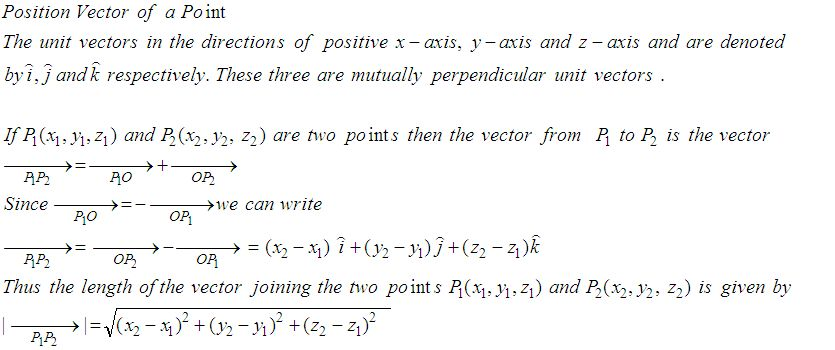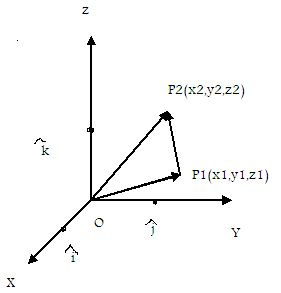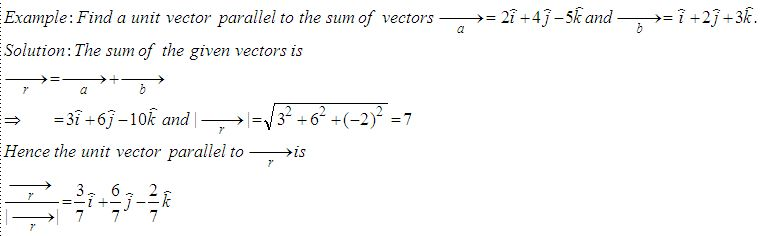Email us to get an instant 20% discount on highly effective K-12 Math & English kwizNET Programs!

#### Online Quiz (WorksheetABCD)

Questions Per Quiz = 2 4 6 8 10

### High School Mathematics - 25.9 Position Vector of a PointDirections: Answer the following.
 Q 1: Find the vector in the direction of the vector -i+2j+2k that has magnitude 7.-3i+2j7(-i+2j+2k)/3i-j+k Q 2: Find the condition that the vectors a = ki + 3j and b = 4i+kj, (k not equal to 0) are parallel.k = 6k2 = 12k = 0 Q 3: Find the vector with initial point P(6,-2) and terminal point Q(4,-8).6i-9j8i+2j-2i+10j Q 4: If P = 2,4,7 and P2 = (-4, -1, 5) then find the magnitude of vector P1P2.6i+5jų653i+6j Q 5: Find the unit vector in the direction of P(1,2,3) towards Q(4,5,6).6i+5j+2k(i+j+k)/ų38j-2k Q 6: Find a unit vector parallel to the sum of vectors a = 2i+4j-5k, b = i+2j+3k2-i83/7i + 6/7j - 2/7 k8i+4j-3k Q 7: Find the unit vector in the direction of of P(1,2) and Q(4,5).(i+j)/ų23i-2j2i+5j Q 8: Find a unit vector in the direction from P(3,2) towards Q(5,6).4i+52i+3i/ų5 + 2j/ų5 Question 9: This question is available to subscribers only! Question 10: This question is available to subscribers only!

#### Subscription to kwizNET Learning System offers the following benefits:

• Unrestricted access to grade appropriate lessons, quizzes, & printable worksheets
• Instant scoring of online quizzes
• Progress tracking and award certificates to keep your student motivated
• Unlimited practice with auto-generated 'WIZ MATH' quizzes